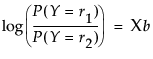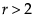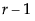Logistic regression fits nominal Y responses to a linear model of X terms. To be more precise, it fits probabilities for the response levels using a logistic function. For two response levels, the function is:where r1 and r2 are the two responses levels, respectively
For r nominal response levels, where, the model is defined bylinear model parameters of the following form:
The fitting principal of maximum likelihood means that the βs are chosen to maximize the joint probability attributed by the model to the responses that did occur. This fitting principal is equivalent to minimizing the negative log-likelihood (–LogLikelihood):

Help created on 9/19/2017